+
Standard Deviation
Central Tendency, Variation, and Distributions
0
of 90 possible points

# Standard Deviation

Author: Sophia Tutorial
##### Description:

Identify how to calculate the standard deviation or variation of a data set.

(more)

Sophia’s self-paced online courses are a great way to save time and money as you earn credits eligible for transfer to many different colleges and universities.*

No credit card required

37 Sophia partners guarantee credit transfer.

299 Institutions have accepted or given pre-approval for credit transfer.

* The American Council on Education's College Credit Recommendation Service (ACE Credit®) has evaluated and recommended college credit for 33 of Sophia’s online courses. Many different colleges and universities consider ACE CREDIT recommendations in determining the applicability to their course and degree programs.

Tutorial
what's covered
This tutorial will explain the concept of standard deviation. Our discussion breaks down as follows:

1. Standard Deviation
2. Calculating Standard Deviation
3. Interpreting Standard Deviation
4. Using Technology

## 1. Standard Deviation

Standard deviation is a measure of variation that we use quite often in statistics. Standard deviation measures spread. You will interpret the standard deviation as the typical amount that you would expect data to be within the mean.

The full name for standard deviation is "standard deviation from the mean." If you break that down, "standard" just means it's typical, "deviation" means that you expect it to be off from the mean, just by chance. So "standard deviation from the mean" states that the data will be away from the mean.

Here is what the formula to find the standard deviation of a sample looks like:

formula
Standard Deviation of a SampleAs complicated as the formula looks, it's actually the one preferred most, provided that the distribution that you’re looking for is roughly symmetric and doesn’t have outliers. If the data is not symmetric or has outliers, you will use a different measure of spread, the interquartile range.

term to know

Standard Deviation
A typical amount by which we would expect a data point to differ from the mean. Typically, about half to two-thirds of the data points fall within one standard deviation of the mean.

## 2. Calculating Standard Deviation

When calculating the formula for standard deviation, you follow these steps:

step by step

Step 1: Subtract the mean from each value.
Step 2: Square those values, resulting in (x minus mean)2.
Step 3: Use the sigma notation, which is the same as summation notation, to add these values.
Step 4: Divide that sum by (n minus 1).
Step 5: Take the square root.EXAMPLE

These are the heights of the Chicago Bulls basketball team.

Height of Chicago Bulls Players
Omer Asik 84
Carlos Boozer 81
Ronnie Brewer 79
Jimmy Butler 79
Luol Deng 81
Taj Gibson 81
Richard Hamilton 79
Mike James 74
Kyle Korver 79
John Lucas III 71
Joakim Noah 83
Derrick Rose 75
Brian Scalabrine 81
Marquis Teague 74
C.J. Watson 74

When considering standard deviation, each of these items is a Xᵢ (X sub 1) in the original formula. Let's follow the five steps to calculate the standard deviation:

step by step

Step 1: The first step is to subtract the mean, which in this case, means you have to first calculate the mean. A thorough explanation of how to calculate the mean is covered in another tutorial, so for today, know that the mean is around 78.33 inches. Therefore, subtract 78.33 from each of these height values.

84 minus 78.33 is 5.67, 81 minus 78.33 is 2.67, etc.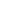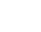84 5.67
81 2.67
79 0.67
79 0.67
81 2.67
81 2.67
79 0.67
74 -4.33
79 0.67
71 -7.33
83 4.67
75 -3.33
81 2.67
74 -4.33
74 -4.33

Step 2: Square those values, resulting in (x minus mean)2.

5.672 is 32.15, 2.672 is 7.13, etc.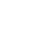84 5.67 32.15
81 2.67 7.13
79 0.67 0.45
79 0.67 0.45
81 2.67 7.13
81 2.67 7.13
79 0.67 0.45
74 -4.33 18.75
79 0.67 0.45
71 -7.33 53.73
83 4.67 21.81
75 -3.33 11.09
81 2.67 7.13
74 -4.33 18.75
74 -4.33 18.75

Step 3: Use this sigma notation, which is the same as summation notation, to add these values up. They sum up to 205.35.84 5.67 32.15
81 2.67 7.13
79 0.67 0.45
79 0.67 0.45
81 2.67 7.13
81 2.67 7.13
79 0.67 0.45
74 -4.33 18.75
79 0.67 0.45
71 -7.33 53.73
83 4.67 21.81
75 -3.33 11.09
81 2.67 7.13
74 -4.33 18.75
74 -4.33 18.75
Sum 205.35

Step 4: Divide that sum by n minus 1. In this case, n is 15 because there were 15 players; therefore n minus 1 equals 14. Dividing our sum by 14 equals 14.67.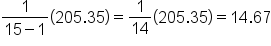hint
If you stopped here, this 14.67 number would measure another kind of variation called "variance." Variance is equivalent to the square of the standard deviation. Variance isn’t used very often, mainly because it is still a squared value. The measurement for variance, in this case, would be 14.67 inches squared, versus inches.

formula
Variance of a Sample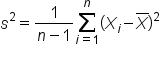Step 5: The final step is to take the square root of that number, which equals 3.83.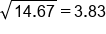Here is an overview of the entire calculation:84 5.67 32.1581 2.67 7.13 79 0.67 0.45 79 0.67 0.45 81 2.67 7.13 81 2.67 7.13 79 0.67 0.45 74 -4.33 18.75 79 0.67 0.45 71 -7.33 53.73 83 4.67 21.81 75 -3.33 11.09 81 2.67 7.13 74 -4.33 18.75 74 -4.33 18.75 Sum 205.35

For this data set, you would expect a good portion of the heights to be within 3.83 inches of the mean, 78.33.

big idea
Variance and standard deviation share an important relationship. The variance is the square of the standard deviation, and the standard deviation is the square root of the variance.

term to know

Variance
The square of standard deviation. While it has some uses in statistics, it is not a practical unit of measurement. It is calculated the same way as standard deviation, but without the square root.

## 3. Interpreting Standard Deviation

Standard deviation is a typical amount by which we would expect values to vary around the mean.

try it
Considering the original list of heights, which fall into one standard deviation of the mean, meaning their heights are either 3.83 above or below 78.33?

Height of Chicago Bulls Players
Omer Asik 84
Carlos Boozer 81
Ronnie Brewer 79
Jimmy Butler 79
Luol Deng 81
Taj Gibson 81
Richard Hamilton 79
Mike James 74
Kyle Korver 79
John Lucas III 71
Joakim Noah 83
Derrick Rose 75
Brian Scalabrine 81
Marquis Teague 74
C.J. Watson 74

You'll notice about ⅔ of the players had heights in this range. That is how you interpret the standard deviation.

## 4. Using Technology

As you may have noticed, calculating the standard deviation can require many steps, which could result in calculation errors. Because of this, the standard deviation is almost always found on a calculator or a spreadsheet, or some kind of applet on the internet. Typically, it is not solved by hand.

If you're frustrated with the calculation you just practiced, you can use your calculator or a spreadsheet in the future.

Here is how to use spreadsheets to calculate standard deviation:

step by step
Step 2: For the formula, type “=stdev(”.
Step 3: Highlight the list that you want to find the standard deviation for, close the parentheses, and hit "Enter."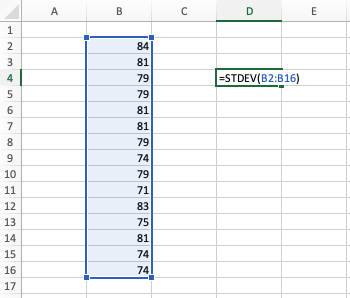This spreadsheet formula finds the same number as the calculation you did by hand: 3.83.

big idea
If you have Excel or another spreadsheet program, there should be a standard deviation formula that you can use.

summary
Interpreting standard deviation is important, but it can be difficult to calculate. For this reason, calculating this number is typically done using technology. It's a measure of how far we would expect a typical data point to be from the mean. Standard deviation is the square root of a value called variance, which is a little bit easier to calculate but not as useful as a standard deviation number. Since the standard deviation is based on the mean, the standard deviation should only be reported as the measure of spread when you're reporting the measure of center to be the mean. You shouldn't mix and match the standard deviation with the median or the interquartile range.

Good luck!

Source: Adapted from Sophia tutorial by Jonathan Osters.

Terms to Know
Standard Deviation

A typical amount by which we would expect a data point to differ from the mean. Typically, about half to two-thirds of the data points fall within one standard deviation of the mean.

Variance

The square of standard deviation. While it has some uses in statistics, it is not a practical unit of measurement. It is calculated the same way as standard deviation, but without the square root.

Formulas to Know
Standard Deviation of a SampleVariance of a SampleRating